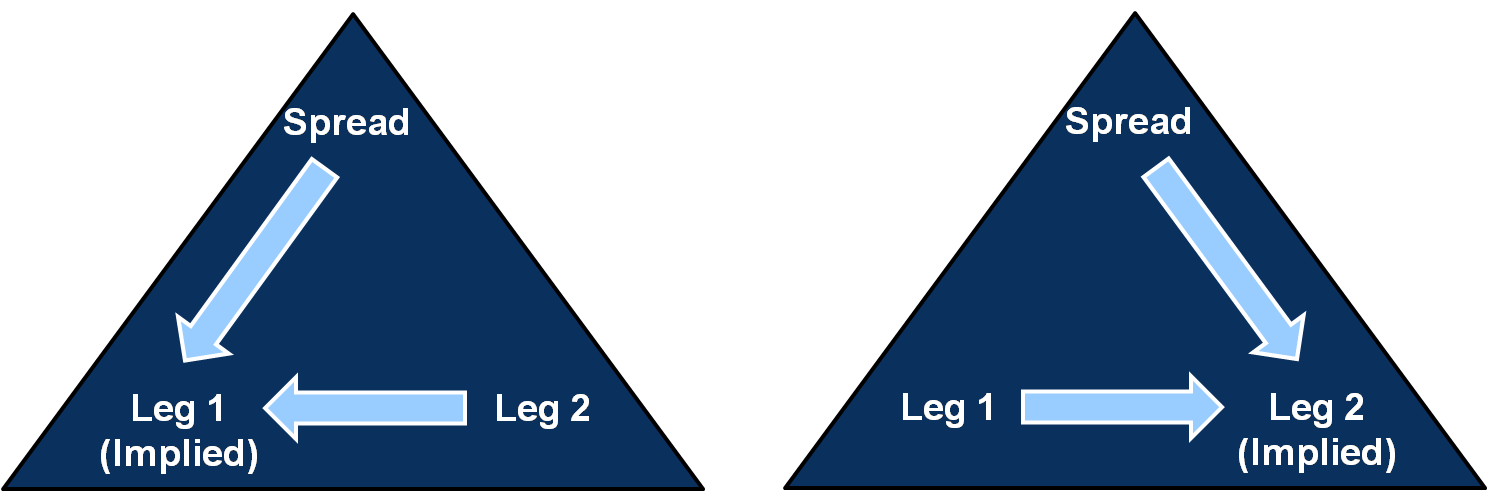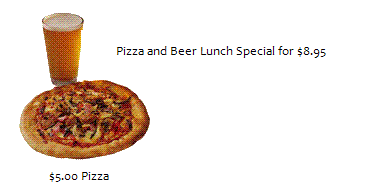← Back to X_TRADER® Help Library

#### Calculating Implied Outs

You are viewing X_TRADER Version 7.17 and higher. For earlier versions, click here

When calculating Implied Outs, a leg price is generated by the spread price and one of the legs. The differential of our known values (the spread price and a leg price) goes into generating our unknown value (a leg price).

Tip: The leg price comes out of the spread.Example: We have two variables in our implied out example: the lunch special price and the price of the pizza.Taking a look at our example, we know that...

• The price of the lunch special is \$8.95 and the price of the pizza is \$5.00.
• Our unknown variable is the price of the beer.

In this example, we have two known values (the cost of the lunch special and the cost of the pizza). We need to calculate for a third value (i.e., the cost of the beer).

Lunch Special

-

Pizza

=

Beer

\$8.95

-

\$5.00

=

\$3.95

Direct Price

Direct Price

Implied Price

(Outright)

(Outright)

The cost of the two known values implies the cost of the third value (i.e., the beer).

Example: This example illustrates how to calculate an Implied Out for the Leg 1 Bid price. In other words, the unknown is the Leg 1 Bid price.

To derive an implied Leg 1 price, take the...

x - Leg 2 = Spread Price

Gold ContractBid PriceAsk Price
Leg 1September6500
Leg 2December6509

Tip: Work back from the spread price using the known leg price.

x

-

6509

=

-9

x

=

6500

Taking a look at the market conditions in our example, we know that...

• The spread bid price is -9. What is the order trying to do? The order is trying to buy September and sell December.
• The spread order can sell December at 6509.
• We need a bid in September to complete the spread order.
• This implies that September must be bought at 6500 to complete the order.

Example: This example illustrates how to calculate an Implied Out for the Leg 2 Bid price.

To derive the implied Leg 2 price, take the...

Leg 1 - x = Spread Price

Gold ContractBid PriceAsk Price
Leg 1March6400
Leg 2June6410
SpreadMarch - June -10

Tip: Work back from the spread price using the known leg price.

6400

-

x

=

-10

x

=

6410

In this example, the unknown is the Leg 2 Bid price. Taking a look at the market conditions in our example, we know that...

• The spread is bid at -10. What is the order trying to do? The order is trying to sell March and buy June.
• The spread order can sell March at 6400.
• We need a bid in June to complete the spread order.
• This implies that June must be bought at 6410 to complete the order.

The Implied Out is a leg price. When calculating Implied Outs, it is important to work back from the spread price using the known leg price.

• If the spread order is to buy the spread and we know either the Ask price of Leg 1 or the Bid price of Leg 2, the market will imply the unknown leg.
• If the spread order is to sell the spread and we know either the Bid price of Leg 1 or the Ask price of Leg 2, the market will imply the unknown leg.